You might like to look at my Geometry book
Some illustrations of theorems in Geometry

Pythagoras' Theorem.
The square on the hypotenuse of a right triangle is equal to the sum of the squares on the other two sides.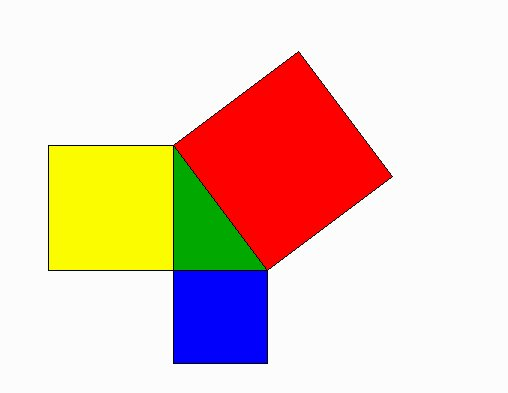Euclid's proof:Another proof: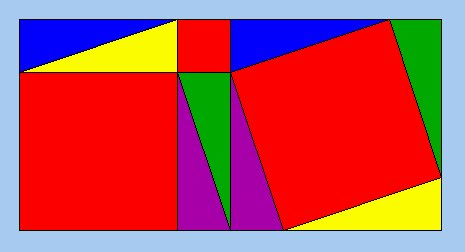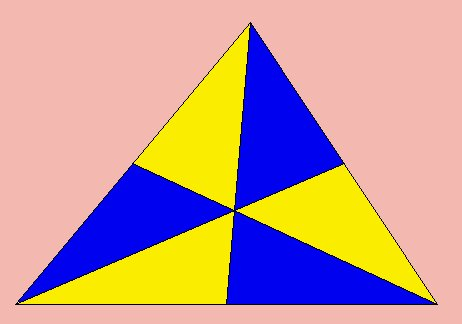The medians of a triangle are concurrent at the centroid.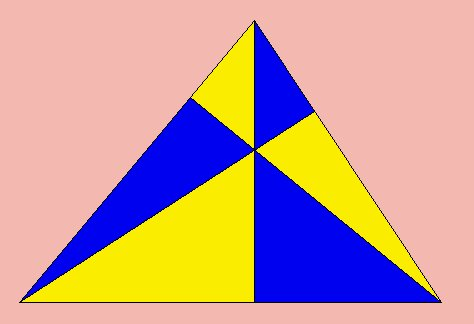The altitudes of a triangle are concurrent at the orthocentre.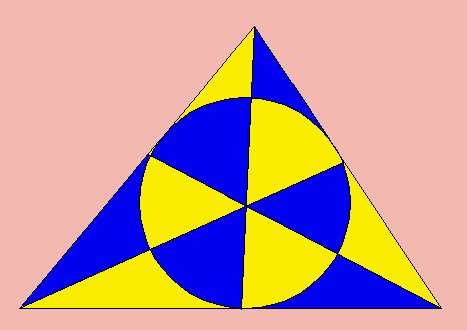The internal bisectors of the angles of a triangle are concurrent at the centre of the incircle.The perpendicular bisectors of the sides of a triangle are concurrent at the centre of the circumcircle.

Pappus' Theorem: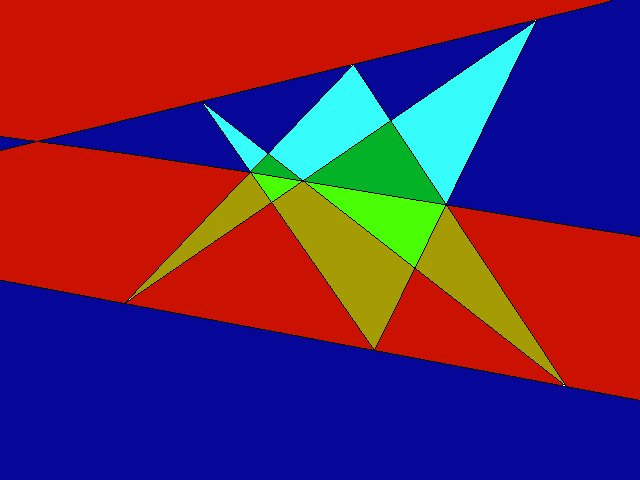Pascal's Theorem:The Simson Line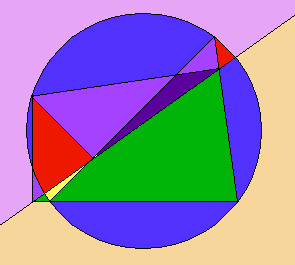The Nine-Point CircleThe Euler LineMorley's Theorem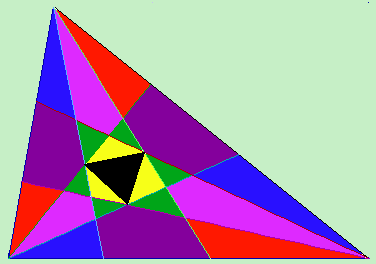The "outer" internal angle trisectors of a triangle intersect to form an equilateral triangle.

Napoleon's Theorem